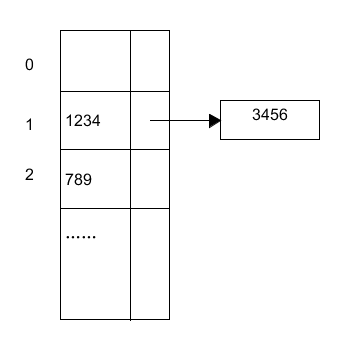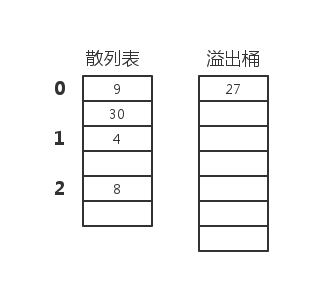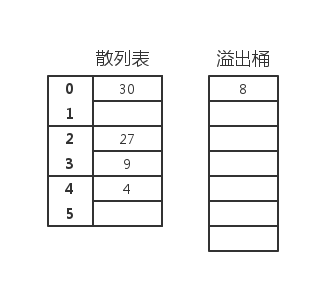# 数据结构：散列（hashing）

## 散列函数

### 平方取中方法

4567 * 4567 = 20857489

### 折叠方法

int h(char* str)
{
int sum, i;
for(sum = i = 0; str[i] != '\0'; ++i)
sum += static_cast<int>(str[i]);
return sum % M;
}

### 将字符串解释为无符号整型和的散列方法

int sfold(char* str)
{
unsigned int sum = 0;
unsigned int* intKey = static_cast<decltype(intKey)>(str);
int len = strlen(str)/4;
for(int i =0;i<len;++i)
sum += intKey[i];

int extra = strlen(str)-len*4;
char temp;
intKey = static_cast<decltype(intKey)>(temp);//or reinterpret_cast?
intKey = 0;
for(int i =0;i<extra;++i)
temp[i] = str[len*4+i];
sum += intKey;
return sum%M;
}

## 开散列方法int h(const Key& k) const
{
return k*7%M;
}

## 闭散列方法

### 桶式散列9 30 27 4 8

int h(int i)
{
return i%B;
}int h(int i)
{
return i%M;
}

### 线性探查

int p(int k, int i)
{
return a*i+b;
}

return (h(k)+p(k, i))%M;

9 30 27 4 8

3 4 5 0 1 2

### 二次探查

int p(int k, int i)
{
return a*i*i+b*i+c;
}

### 双散列方法

int p(int k, int i)
{
return i*h2(k);
}

h2 是第二个散列函数

## 删除

1. 删除不应当影响后面的检索。
2. 删除的槽应当可以为后来的插入操作所使用。

–END–